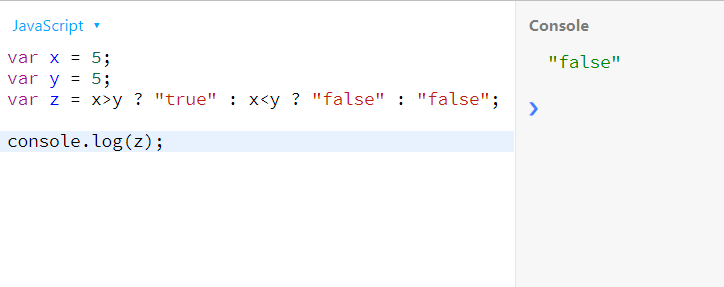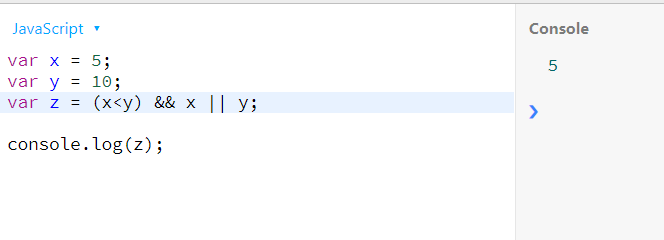# JavaScript 中的内联 if 语句

## JavaScript 中带有三元运算的简单内联 `if` 语句

``````var x = 5;
var y = 10;
var z = x>y ? x : y;

console.log(z);
``````## JavaScript 中的多条件内联 `if` 语句三元运算

``````var x = 5;
var y = 5;
var z = x>y ? "true" : x<y ? "false" : "false";

console.log(z);
``````## JavaScript 中带有逻辑运算符的内联 `if` 语句

``````var x = 5;
var y = 10;
var z = (x<y) && x || y;

console.log(z);
``````## JavaScript 中带有三元运算的简单内联 `if` 语句

``````var x = 5;
var y = 10;
var z = x>y ? x : y;

console.log(z);
``````## JavaScript 中的多条件内联 `if` 语句三元运算

``````var x = 5;
var y = 5;
var z = x>y ? "true" : x<y ? "false" : "false";

console.log(z);
``````## JavaScript 中带有逻辑运算符的内联 `if` 语句

``````var x = 5;
var y = 10;
var z = (x<y) && x || y;

console.log(z);
``````## JavaScript 中带有三元运算的简单内联 `if` 语句

``````var x = 5;
var y = 10;
var z = x>y ? x : y;

console.log(z);
``````## JavaScript 中的多条件内联 `if` 语句三元运算

``````var x = 5;
var y = 5;
var z = x>y ? "true" : x<y ? "false" : "false";

console.log(z);
``````## JavaScript 中带有逻辑运算符的内联 `if` 语句

``````var x = 5;
var y = 10;
var z = (x<y) && x || y;

console.log(z);
``````